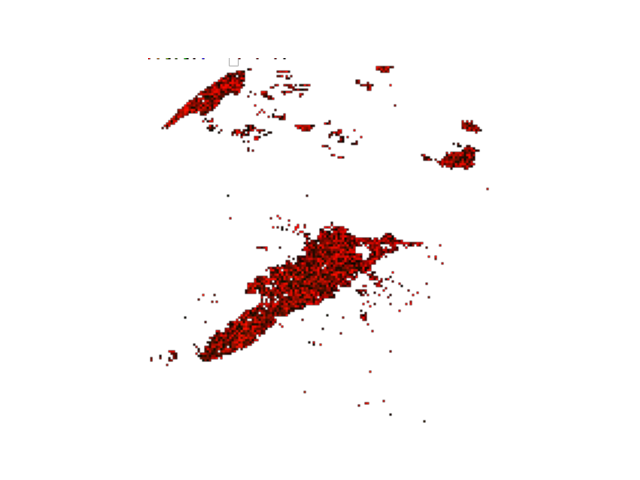# Convert to RGB format (vector data)¶

This example demonstrates convertion from wind data to uv rgb format (with HKO earth format).

## Definitions¶

Import all required modules and methods:

# Python package to allow system command line functions
import os
# Python package to manage warning message
import warnings
# Python package for time calculations
import pandas as pd
# Python package for numerical calculations
import numpy as np
# Python package for image generation
from PIL import Image
# Python package for image preview
import matplotlib.pyplot as plt

# swirlspy iris parser function
# swirlspy regrid function
from swirlspy.preprocess import grid_align
# swirlspy rgb convertion function
from swirlspy.utils.conversion import to_rgb_data, to_hko_earth_format
# directory constants
from swirlspy.tests.samples import DATA_DIR
from swirlspy.tests.outputs import OUTPUT_DIR

warnings.filterwarnings("ignore")

# Logging
start_time = pd.Timestamp.now()


Out:

## You are using the Python ARM Radar Toolkit (Py-ART), an open source
## library for working with weather radar data. Py-ART is partly
## supported by the U.S. Department of Energy as part of the Atmospheric
## Radiation Measurement (ARM) Climate Research Facility, an Office of
## Science user facility.
##
## If you use this software to prepare a publication, please cite:
##
##     JJ Helmus and SM Collis, JORS 2016, doi: 10.5334/jors.119


# Specify the basetime
basetime = pd.Timestamp('201902190800')

os.path.join(
DATA_DIR,
basetime.strftime("iris/ppi/TMS%y%m%d%H%M02.PPIMK3B")
)
)

initialising_time = pd.Timestamp.now()


## Reproject to WGS 84, required for HKO earth format¶

# calculate x, y step size
y_step = -0.025
x_step = 0.025
y = np.arange(24.5, 20.5, y_step)
x = np.arange(112, 116, x_step)

reflec_wgs84 = grid_align(
reflec, reflec.attrs['area_def'].proj_str,
x, y, '+proj=longlat +datum=WGS84 +no_defs'
)

preparation_time = pd.Timestamp.now()


## Convert image data into rgb format¶

# only shape with (y, x) is allowed
data = reflec_wgs84.sel(time=basetime)

# only allow positive values
data = data.where(data >= 0)

# this step is not necessary, depends on any meta data and preprocess is required by your platform
earth_data = to_hko_earth_format(data, coords_dp=3, y_step=y_step, x_step=x_step)
rgb = to_rgb_data(earth_data)

convertion_time = pd.Timestamp.now()


## Visualisation¶

path = os.path.join(OUTPUT_DIR, "rgb_wind.png")
with Image.fromarray(rgb, 'RGBA') as img:
img.save(path, 'png')

# preview
with Image.open(path) as image:
plt.axis('off')
plt.imshow(image)
plt.plot()
plt.show()

visualise_time = pd.Timestamp.now()## Checking run time of each component¶

print(f"Start time: {start_time}")
print(f"Initialising time: {initialising_time}")
print(f"Preparation time: {preparation_time}")
print(f"Convertion time: {convertion_time}")
print(f"Visualise time: {visualise_time}")

print(f"Time to initialise: {initialising_time - start_time}")
print(f"Time to prepare information: {preparation_time - initialising_time}")
print(f"Time to convertion: {convertion_time - preparation_time}")
print(f"Time to visualise: {visualise_time - convertion_time}")

print(f"Total: {visualise_time - start_time}")


Out:

Start time: 2021-11-29 08:54:12.671812
Initialising time: 2021-11-29 08:54:12.690067
Preparation time: 2021-11-29 08:54:12.841664
Convertion time: 2021-11-29 08:54:12.848889
Visualise time: 2021-11-29 08:54:13.064131
Time to initialise: 0 days 00:00:00.018255
Time to prepare information: 0 days 00:00:00.151597
Time to convertion: 0 days 00:00:00.007225
Time to visualise: 0 days 00:00:00.215242
Total: 0 days 00:00:00.392319


Total running time of the script: ( 0 minutes 1.060 seconds)

Gallery generated by Sphinx-Gallery